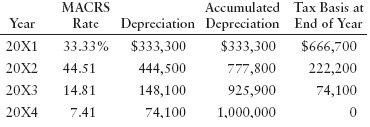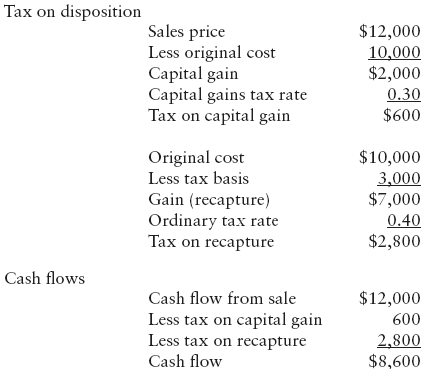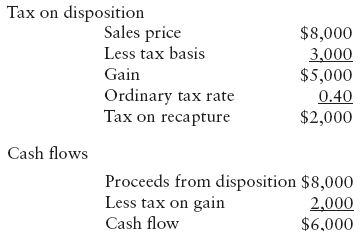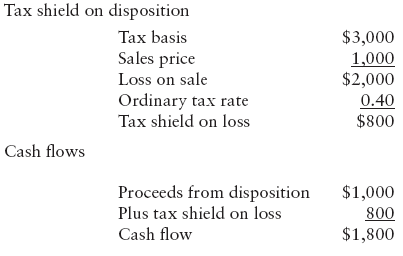Connect with us

# Asset Disposals, Cash Flow and Their Tax ConsequencesPublished

onInvestment cash flows takes into account asset acquisition costs and asset disposal costs. The analysis of an investment must consider all the cash flows associated with acquiring and disposing of assets. in any project, there are a number of different types of investment cash flows to consider, including the cost of acquisition (e.g., cost of the asset, setup expenditures, including shipping and installation, and any tax credit). At the end of the useful life of an asset, the company may be able to sell the asset or may have to pay another party to haul it away or close it down. If the company is making a decision that involves replacing an existing asset, the cash flow from disposing of the old asset must be considered because it is a cash flow relevant to the acquisition of the new asset. If a company disposes of an asset, whether at the end of its useful life or when it is replaced, two types of cash flows must be considered: what the company expects to receive or expects to pay in disposing of the asset and any tax consequences resulting from the disposal. This post discuss asset disposition and the two type of cash flow includes its tax consequences.

The proceeds are what the company expects to sell the asset for if sold. If the company must pay for the disposal of the asset, this cost is a cash outflow.

Cash low from disposing assets = Proceeds or payment from disposing assets – taxes from disposing assets

Consider the investment in a gas station. The current owner may want to leave the business (retire, whatever), selling the station to another gas station proprietor. but if a buyer cannot be found because of lack of gas buyers in the area, the current owner may be required to remove the underground gasoline storage tanks to prevent environmental damage. Thus, a cost is incurred at the end of the asset’s life.

The tax consequences are a bit more complicated. Taxes depend on the expected sales price and the tax basis at the time of disposition:

• If a company sells the asset for more than its tax basis but less than its original cost; the difference between the sales price and the tax basis is a gain, taxable at ordinary tax rates.
• If the company sells the asset for more than its tax basis but less than its original cost: the difference between the sales price and the tax basis is a gain, taxable at ordinary tax rates.
• If a company sells the asset for more than its original cost: then the gain is broken into two parts: the capital gain (the difference between the sales price and the original cost) and recapture of depreciation (the difference between the original cost and the tax basis).

The capital gain is the benefit from the appreciation in the value of the asset and may be taxed at special rates, depending on the tax law at the time of sale. The recapture of depreciation represents the amount by which the company has over-depreciated the asset during its life. This means that more depreciation has been deducted from income (hence reducing taxes) than necessary to reflect the actual depreciation of the asset. The recapture portion is taxed at the ordinary tax rates, because this excess depreciation taken all these years has reduced taxable income.

• If a company sells an asset for less than its tax basis: the result is a capital loss. in this case, the asset’s value has decreased by more than the amount taken for depreciation for tax purposes. a capital loss is given special tax treatment:
• If there are capital gains in the same tax year as the capital loss: the gains and losses are combined, so that the capital loss reduces the taxes paid on capital gains.
• If there are not sufficient capital gains to offset against the capital loss: the remaining capital loss is used to reduce ordinary taxable income.

The benefit from a loss on the sale of an asset is the amount by which taxes are reduced. The reduction in taxable income is referred to as a tax shield because the loss shields some income from taxation. For example: if a company has a loss of \$1,000 on the sale of an asset and has a tax rate of 40%, this means that its taxable income is \$1,000 less and its tax liability is \$400 less than it would have been without the sale of the asset.

Suppose a company is evaluating an asset that costs \$10,000 that it expects to sell in five years. Suppose further that the tax basis of the asset for tax purposes will be \$3,000 after five years and that the company’s tax rate is 40%. What are the expected cash lows from disposing of this asset?

If the company expects to sell the asset for \$8,000 in five years, \$10,000 – \$3,000 = \$7,000 of the asset’s cost will be depreciated; yet the asset lost only \$10,000 – \$8,000 = \$2,000 in value. therefore, the company has over-depreciated the asset by \$5,000. Because this over-depreciation represents deductions to be taken on the company’s tax returns over the five years that don’t reflect the actual depreciation in value (the asset doesn’t lose \$7,000 in value, only \$2,000), this \$5,000 is taxed at ordinary tax rates. If the company’s tax rate is 40%, the tax will be 40% × \$5,000 = \$2,000.

The cash low from disposition is the sum of the direct cash low (someone pays us for the asset or the company pays someone to dispose of it) and the tax consequences. In this example, the cash low is the \$8,000 we expect someone to pay the company for the asset, less the \$2,000 in taxes we expect the company to pay, or \$6,000 cash inflow.

Suppose instead that the company expects to sell this asset in ive years for \$12,000. Again, the asset is over-depreciated by \$7,000. In fact, the asset is not expected to depreciate, but rather appreciate over the ive years. the \$7,000 in depreciation is recaptured after ive years and taxed at ordinary rates: 40% of \$7,000, or \$2,800. The \$2,000 capital gain is the appreciation in the value of the asset and may be taxed at special rates. if the tax rate on capital gain income is assumed to be 30%, the company would expect to pay 30% of \$2,000, or \$600 in taxes on this gain. Selling the asset in five years for \$12,000 therefore results in an expected cash inflow of \$12,000 – \$2,800 – \$600 = \$8,600.

Suppose the company expects to sell the asset in five years for \$1,000. if the company can reduce its ordinary taxable income by the amount of the capital loss, \$3,000 – \$1,000 = \$2,000, its tax liability will be 40% of \$2,000, or \$800 because of this loss.

As mentioned earlier, this reduction in the taxes is a tax shield, since the loss “shields” \$2,000 of income from taxes. Combining the \$800 tax reduction with the cash low from selling the asset, the \$1,000, gives the company a cash inflow of \$1,800. The calculation of the cash low from disposition for the alternative sales prices of \$8,000, \$12,000, and \$1,000 will be discussed at the end of this post.

### Disposal of New Investment

If a company sells the asset for more that its original cost, then the gain is broken into two parts: a capital gain, which is the difference between the sales price and the original cost; and recapture depreciation, which is the difference between the original cost and the tax basis. The “capital gain” is the benefit from the appreciation in the value of the asset and may be taxed at special rates, depending on the tax law at the time of sale.

The recapture portion is taxed at the ordinary tax rates, since this excess depreciation. Taken all these years has reduced taxable income.

If a company sells an asset for less than its tax basis, the result is a capital loss. In this case, the asset’s value has decreased by more than the amount taken for depreciation for tax purposes. A capital loss is given special tax treatment as discussed earlier. The benefit from a loss on the sale of an asset is the amount by which taxes are reduced (i.e., the tax shield). if the company has a loss of \$1,000 on the sale of an asset and has a tax rate of 40%, this means that its taxable income is \$1,000 less and its taxes are \$400 less than they would have been without the sale of the asset.

The relevant book value of an asset in the case of determining the tax gain or loss—and hence the related cash low—is the tax basis of the asset. The tax basis is the difference between the original cost of the asset and any accumulated depreciation, where this accumulated depreciation is the prescribed depreciation according to the tax code.

Consider an asset purchased at the beginning of 20X1 for \$1 million. If this is a 3-year MACRS asset, its depreciation, accumulated depreciation, and tax basis each year is the following:MACRS depreciation rates are the rates prescribed by the U.S. Internal Revenue Code for depreciation for tax purposes.

Whether there is a gain or a loss on the sale of this asset depends on whether the sales prices is above the tax basis (hence, a gain), or below the tax basis (hence, a loss). If the sales price is above the original cost of \$1 million, the total gain is broken into two parts—that which is above the original cost (sales price – original cost), which is taxed at capital gains rates, and that which is below (original cost – tax basis), which is taxed at ordinary rates.

What is the gain or loss if the asset described above is sold:

(a) at the end of 20X2, for \$250,000?

Gain = \$250,000 – \$222,200 = \$27,800. This gain is taxed at ordinary rates because the sales price of \$250,000 is less than original cost of \$1 million.

(b) at the end of 20X3, for \$50,000?

Loss = \$50,000 – \$74, 00 = –\$24, 00. This is a capital loss because it is sold at less than the tax basis at the time of the sale.

c) at the end of 20X4, for \$ , 00,000?

Gain = \$ , 000,000 – \$666,700 = \$433,300

The gain is taxed in two parts:

• \$100,000 taxed as a capital gain
• \$333,300 taxed at ordinary rates

### Disposal of Existing Asset (s)

Let’s also not forget about disposing of any existing assets. Suppose the company bought equipment 10 years ago and at that time expected to be able to sell it 5 years later for \$ 10,000. If the company decides today to replace this equipment, it must consider what it is giving up by not disposing of an asset as planned.

If the company does not replace the equipment today, it would continue to depreciate it for five more years and then sell it for \$10,000. If the company replaces the equipment today, it would not have five more years’ depreciation on the replaced equipment and it would not have \$10,000 in five years (but perhaps some other amount today). This \$10,000 in five years, less any taxes, is a forgone cash low that we must figure into the investment cash lows. also, the depreciation the company would have had on the replaced asset must be considered in analyzing the replacement asset’s operating cash lows. so, any time that a company is making a decision pertaining to replacing an asset, the forgone depreciation must be accounted for.

### Expected Cash Flows from an Asset Disposal [Case Examples]

Suppose a company is evaluating an asset that costs \$ 0,000 that it expects to sell in ive years. Suppose further that the tax basis of the asset will be \$3,000 after five years and that the company’s tax rate is 40%. What are the expected cash lows from disposing of this asset?

Case 1: Sell the asset for \$8,000 [Original cost > Expected sales price > Tax basis]

If the company expects to sell the asset for \$8,000 in five years, \$10,000 – \$3,000 = \$7,000 of the asset’s cost will be depreciated, yet the asset lost only \$10,000 – \$8,000 = \$2,000 in value. Therefore, the company has over-depreciated the asset by \$5,000. since this over-depreciation represents deductions to be taken on the company’s tax returns over the five years that don’t reflect the actual depreciation in value (the asset doesn’t lose \$7,000 in value, only \$2,000), this \$5,000 is taxed at ordinary tax rates. If the company’s tax rate is 40%, the tax = 40% × \$5,000 = \$2,000.

The cash low from disposition is the sum of the direct cash low (someone pays the company for the asset or the company pays another party to dispose of it) and the tax consequences. In this example, the cash low is the \$8,000 the company expects someone to pay for the asset, less the \$2,000 in taxes the company expects to pay, or \$6,000 cash inflow.Case 2: Sell the asset for \$12,000 [Expected sales price > Original cost > Tax basis]

Suppose instead that the company expects to sell this asset in five years for \$12,000. Again, the asset is over-depreciated by \$7,000. in fact, the asset is not expected to depreciate, but rather appreciate over the five years. The \$7,000 in depreciation is recaptured after five years and taxed at ordinary rates: 40% of \$7,000, or \$2,800. the \$2,000 capital gain is the appreciation in the value of the asset and may be taxed at special rates. Assuming the tax rate on capital gain income is 30%, the company expects to pay 30% of \$2,000, or \$600 in taxes on this gain. Selling the asset in five years for \$12,000 therefore results in an expected cash inflow of \$12,000 – 2,800 – 600 = \$8,600.Case 3: Sell the asset for \$1,000 [Tax basis > Expected sales price]

Suppose the company expects to sell the asset in ive years for \$1,000. if the company can reduce its ordinary taxable income by the amount of the capital loss, \$3,000 – 1,000 = \$2,000, its tax liability will be 40% of \$2,000, or \$800 because of this loss. the loss “shields” \$2,000 of income from taxes. Combining the \$800 tax reduction with the cash low from selling the asset, the \$1,000, gives the company a cash inflow of \$1,800.Are you looking for easy accounting tutorial? Established since 2007, Accounting-Financial-Tax.com hosts more than 1300 articles (still growing), and has helped millions accounting student, teacher, junior accountants and small business owners, worldwide.